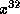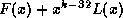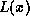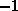Software Implementations# Software Implementations

In all cases, the macro QUOTIENT has the value 0x04c11db7. Although I am detailing these in terms of software, they have direct parallel implementations in the hardware domain. I am merely describing them from the point of view of a programmer implementing them in software.

## Algorithm One

The first and most obvious algorithm is to simply implement the hardware algorithm in software. We use a 32 bit register to store the current remainder. For each byte in the message we take each bit in turn and if a new term inis generated we XOR in the polynomial. The code is as follows:

```unsigned int wombat(unsigned char *data, int len)
{
unsigned int        result;
int                 i,j;
unsigned char       octet;

result = -1;

for (i=0; i<len; i++)
{
octet = *(data++);
for (j=0; j<8; j++)
{
if ((octet >> 7) ^ (result >> 31))
{
result = (result << 1) ^ QUOTIENT;
}
else
{
result = (result << 1);
}
octet <<= 1;
}
}

return ~result;             /* The complement of the remainder */
}```

The user must be careful to write the four octets of the result into the data part of the cell in the correct order (that is bits 24-31 first and bits 0-7 last) irrespective of the endian of the machine.

## Algorithm two

The next algorithm makes the observation that it is possible to do the calculation by multiplying byat the end by feeding in an additional 32 zeros, rather than by doing it at the beginning of the division. We initialise the register to the first thirty two bits of.

The code is written assuming that the transmitting end calls with the length field higher by an additional four bytes (which are where the CRC will be put and which are initially zero). The code is as follows:

```unsigned int wombat(unsigned char *data, int len)
{
unsigned int        result;
int                 i,j;
unsigned char       octet;

if (len < 4) abort();

result = *data++ << 24;
result |= *data++ << 16;
result |= *data++ << 8;
result |= *data++;
result = ~ result;
len -=4;

for (i=0; i<len; i++)
{
octet = *(data++);
for (j=0; j<8; j++)
{
if (result & 0x80000000)
{
result = (result << 1) ^ QUOTIENT ^ (octet >> 7);
}
else
{
result = (result << 1) ^ (octet >> 7);
}
octet <<= 1;
}
}

return ~result;             /* The complement of the remainder */
}```

This code contains an additional benefit which is that it is possible to perform the computation at the recipient without multiplying by the additionalterm. Thus the remainder in equation (8) is simplyand the calculation function returns 0.

## Algorithm three

Algorithm three is the algorithm which I have seen most usually in other literature and implementations. It is a substantial optimisation based on the observation that in algorithm two, (apart from the first thirty two bits) the data to be transmitted is not needed for the control path until some thirty two bits later. This permits the division by subtraction to be done more than one bit at a time using a pre-generated table.

This table is normally indexed by eight bits because in that case the table is 1K in size. Attempting to do more bits than this at once (e.g. 16) is usually slower because at 256K the table has horrific cache performance.

The code to generate the table is:

```unsigned int crctab;

void crc32_init(void)
{
int i, j;

unsigned int crc;

for (i = 0; i < 256; i++)
{
crc = i << 24;
for (j = 0; j < 8; j++)
{
if (crc & 0x80000000)
crc = (crc << 1) ^ QUOTIENT;
else
crc = crc << 1;
}
crctab[i] = crc;
}
}```

The implementation then proceeds byte wise feeding the eight bit subtraction term in at each stage along with the next eight bits of data. The code is:

```unsigned int wombat(unsigned char *data, int len)
{
unsigned int        result;
int                 i;
unsigned char       octet;

if (len < 4) abort();

result = *data++ << 24;
result |= *data++ << 16;
result |= *data++ << 8;
result |= *data++;
result = ~ result;
len -=4;

for (i=0; i<len; i++)
{
result = (result << 8 | *data++) ^ crctab[result >> 24];
}

return ~result;
}```

## Algorithm four

This code is copyright © 1993 Richard Black. All rights are reserved. You may use this code only if it includes a statement to that effect.

This algorithm takes the observation used to produce algorithm three one stage further. Whereas it still performs the division eight bits at a time for cache performance reasons, it is designed in such a way that the data can be fed into the remainder in thirty two bit units (which are more efficient units on most computers). This necessitates re-ordering the bits of the polynomial in a non-monotonic fashion depending on the endian of the computer on which the algorithm is running. The polynomials in the lookup table likewise have the same non-linear transform applied to them as they are generated.

The code for initialising the table becomes:

```unsigned int crctab;

void crc32_init(void)
{
int i,j;

unsigned int crc;

for (i = 0; i < 256; i++)
{
crc = i << 24;
for (j = 0; j < 8; j++)
{
if (crc & 0x80000000)
crc = (crc << 1) ^ QUOTIENT;
else
crc = crc << 1;
}
crctab[i] = htonl(crc);
}
}```

Note the use of htonl to rearrange the terms in the partial quotients. The calculation code then also becomes dependent on the endian of the machine:

```unsigned int wombat(unsigned char *data, int len)
{
unsigned int        result;
unsigned int        *p = (unsigned int *)data;
unsigned int        *e = (unsigned int *)(data + len);

if (len < 4) abort();

result = ~*p++;

while( p<e )
{
#if defined(LITTLE_ENDIAN)
result = crctab[result & 0xff] ^ result >> 8;
result = crctab[result & 0xff] ^ result >> 8;
result = crctab[result & 0xff] ^ result >> 8;
result = crctab[result & 0xff] ^ result >> 8;
result ^= *p++;
#else
result = crctab[result >> 24] ^ result << 8;
result = crctab[result >> 24] ^ result << 8;
result = crctab[result >> 24] ^ result << 8;
result = crctab[result >> 24] ^ result << 8;
result ^= *p++;
#endif
}

return ~result;
}```

Of course this now only works for word aligned data and assumes that the data is an exact number of words. I do not regard these as significant limitations. This code is approximately twice as fast as algorithm three. It should also be noticed that since the data is not broken up into bytes, this code has an even larger benefit when used as an integral part of a data copy routine. The result is also in the local form and should be written directly as a word to the data and not as a sequence of bytes.

The tinkerer will observe that the C can be made slightly more beautiful by rearranging the insertion of the data to the start of the loop, using a pre-condition of, and stopping early. However, apart from the issue at the receiver, such code will be considerably slower, because the data is required synchronously. In the code as I have written it, the compilerwill perform the load on p early in the loop body, and so the load delay will have passed by the time the data is required.

This loop compiles to 16 instructions on the Arm, 29 on the Mips, 30 on the Alpha, and 19 on the HP-PA. The Arm's instruction count is so low because of the ability to perform shifts for free on all operations, but it looses out on its blocking loads to about the same number of cycle as the Mips. The Alpha gains over the Mips with its s4addq instruction, but looses this win because 32bit loads sign extend and require zeroed. The HP-PA assembler is too weird to comment further.

On all architectures, assuming the 1K lookup table is in the cache, the algorithm proceeds at an average of about 1 bit per clock cycle. This represents 25 Mbit/sec on the FPC3 or Maxine, and 150 Mbit/sec on the Sandpiper.

This implementation is particularly suitable for hardware at a point where the data path is thirty two bits wide. The generating register can be implemented as a 32 bit register with an 8 bit barrel roll operation. Between each data word being exclusive-ored in, four rolls with exclusive or of the quotient can be performed. (or two of sixteen). This makes the xor circuitry much simpler and reduces the percentage of cycles which must involve the actual data.